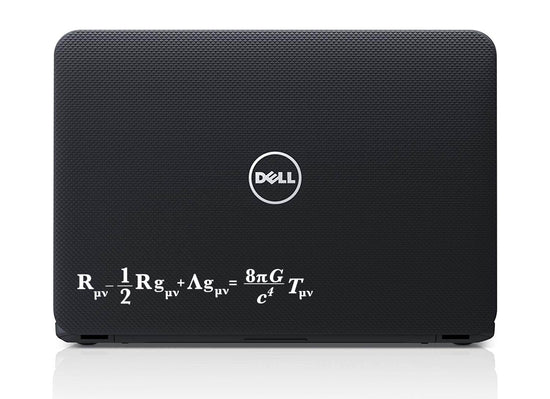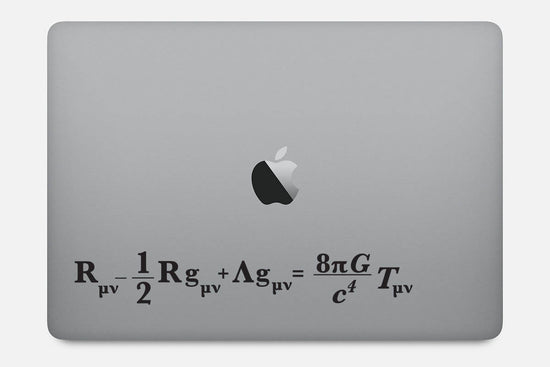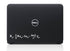Buttered Kat

# Einstein Field Equation for General Relativity Decal Sticker

The EFE is a tensor equation relating a set of symmetric 4 × 4 tensors. Each tensor has 10 independent components. The four Bianchi identities reduce the number of independent equations from 10 to 6, leaving the metric with four gauge fixing degrees of freedom, which correspond to the freedom to choose a coordinate system. Where Rμν is the Ricci curvature tensor, R is the scalar curvature, gμν is the metric tensor, Λ is the cosmological constant, G is Newton's gravitational constant, c is the speed of light in vacuum, and Tμν is the stress–energy tensor.

It can be applied to any smooth surface, comes in two sizes and two colors. Contact us if you require custom size or color.

Proudly Made in the USA.
• Albert Einstein
• chemistry
• Chemistry decal
• computer decal
• computer sticker
• Decal
• equation
• Physics
• Physics Decal
• science
Regular price
\$7.99
Sale price
\$7.99
Regular price

Couldn't load pickup availability

••••••••••••••••